Addition is the oldest activity of mathematics field that is leveraging people since ages. It is famous between successful mathematicians since more than 6000 years. Even counting is the early part of addition. According to the official evidence of addition, Egyptians and Babylonians in 2000 BC. Mathematicians discovered the symbols of additions and subtraction in 16th century. Before that time people write all expressions in words that consumed their lots of time in solving problems. Definition of Addition The process of combining two or more sets of objects into a single set is called addition in general. In mathematics, it is the process of calculating the sum or total of more than two numbers as an arithmetic activity.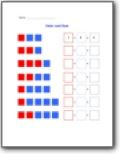###### Color and Sum (Red and Blue) Small

We use red and blue squares to replicate the sums that you need to determine. The two smalls are five or less.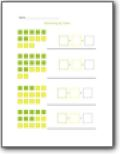###### Color and Sum (Green and Yellow) Small

The same worksheet as the previous, but we switch out the colors to green and yellow.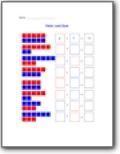###### Color and Sum (Red and Blue) Large

We use red and blue squares to replicate the sums that go up to tens.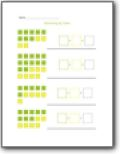###### Color and Sum (Green and Yellow) Large

This worksheet has problems that use colored squares to give students a guide for completing simple math problems.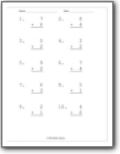Find the sums of these single digits in a vertical manner. The addends are randomly paired.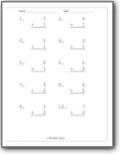Find the sums of these single digits in a vertical manner. The bottom addend values are incrementally increasing by sheet. It starts a 1 and rises to 6.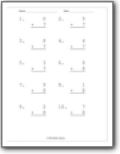Find the sums of these single digits in a vertical manner. The bottom addend values are incrementally increasing by sheet. It starts a 7 and rises to 12.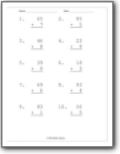###### Single and Double Digit Addition

These problems are setup vertically with the top row being double digits and the bottom value being a single digit.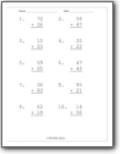Both values are made up of two digits.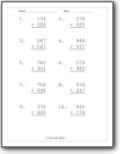Find the sums of two three-digit values.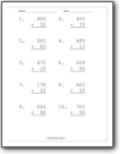###### Double and Triple Digit Addition

Setup in a vertical manner the top addend is a triple digit value and the bottom addend is double digits.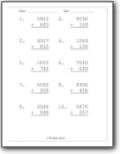These are sums of three and four digit values.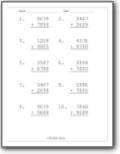These are sums of four digit values.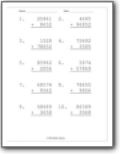###### Five and Four Digit Addition

These problems get a bit lengthy because the values are composed of five and four numbers.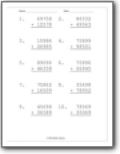Students will find the value of two five digit values.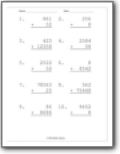###### Mixed Digit Sums

All of these sums are composed of a mixed number of digits.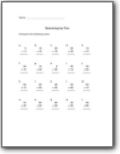###### Summing by Five

Compute the following sums.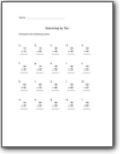###### Summing by Ten

A set of worksheet problems with zero as the ones digit.